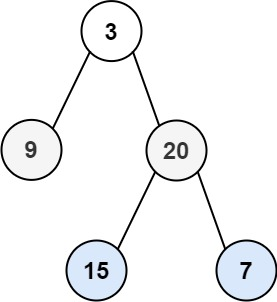# 103. Binary Tree Zigzag Level Order Traversal / Medium

Given the `root` of a binary tree, return the zigzag level order traversal of its nodes’ values. (i.e., from left to right, then right to left for the next level and alternate between).

## Example 1:Input: root = [3,9,20,null,null,15,7]
Output: [,[20,9],[15,7]]

## Example 2:

Input: root = 
Output: []

Input: root = []
Output: []

## Constraints:

• The number of nodes in the tree is in the range `[0, 2000]`.
• `-1000 <= Node.val <= 1000`

# Solution:

## 效能

### Complexity

• Time Complexity: O(N)
• Space Complexity: O(N)

### LeetCode Result

• Runtime: 3 ms
• Memory Usage: 11.8 MB